• java极差算法
千次阅读
2019-01-15 15:58:36
/**
*
*  * @描述:集中趋势量数：极差（不包含） <br/>
*  * @方法名: range <br/>
*  * @param in <br/>
*  * @return <br/>
*  * @返回类型 double <br/>
*  * @创建人 micheal <br/>
*  * @创建时间 2019年1月2日下午10:26:20 <br/>
*  * @修改人 micheal <br/>
*  * @修改时间 2019年1月2日下午10:26:20 <br/>
*  * @修改备注 <br/>
*  * @since <br/ >  * @throws <br/>
*
*/
public static double range(double[] in) {
if (in == null) {
throw new java.lang.NumberFormatException();
}
double max = Double.MIN_VALUE;
double min = Double.MAX_VALUE;
for (int i = 0; i < in.length; i++) {
max = Math.max(max, in[i]);
min = Math.min(min, in[i]);
}
// return max - min;
return Mutil.subtract(max, min);
}

/**
*
*  * @描述: 变异性量数：极差（包含） <br/>
*  * @方法名: range2 <br/>
*  * @param in <br/>
*  * @return <br/>
*  * @返回类型 double <br/>
*  * @创建人 micheal <br/>
*  * @创建时间 2019年1月2日下午10:26:08 <br/>
*  * @修改人 micheal <br/>
*  * @修改时间 2019年1月2日下午10:26:08 <br/>
*  * @修改备注 <br/>
*  * @since <br/>
*  * @throws <br/>
*
*/
public static double range2(double[] in) {
if (in == null) {
throw new java.lang.NumberFormatException();
}
double max = Double.MIN_VALUE;
double min = Double.MAX_VALUE;
for (int i = 0; i < in.length; i++) {
max = Math.max(max, in[i]);
min = Math.min(min, in[i]);
}
// return max - min + 1;
return Mutil.subtract(max, min) + 1;
}

测试代码，打印结果，包含：50.0 ，不包含：51.0

double[] in4 = { 98, 86, 77, 56, 48 };
log.info("计算[极差]：" + range(in4));
log.info("计算[极差]2：" + range2(in4));

更多相关内容
• ## java实现Canny算法

千次阅读 2022-04-26 13:26:12
算法步骤 ①灰度化：常用公式--Gray=0.299R+0.587G+0.114B ②高斯滤波 ③计算梯度值和方向 ④非大值抑制 ⑤双阈值的选取 ⑥滞后边界跟踪

目录

Canny基本思想

算法步骤

高斯滤波

计算梯度值和方向

非极大值抑制

双阈值的选取

滞后边界跟踪

# Canny基本思想

基本思想：寻找图像梯度的局部最大值。
Canny 提出了边缘检测算子优劣评判的三条标准：
① 高检测率。边缘检测算子应该只对边缘进行响应，检测算子不漏检任何边缘，也不应该将非边缘标记为边缘。
② 精确定位。检测到的边缘与实际边缘之间的距离要尽可能的小。
③ 明确的响应。对每一条边缘只有一次响应，只得到一个点。
优点：在一阶微分算子的基础上，增加了非最大值抑制和双阈值。
非极大值抑制：不仅可以有效地抑制多响应边缘，而且还可以提高边缘的定位精度；
双阈值：可以有效减少边缘的漏检率。

# 算法步骤

灰度化：常用公式 -- Gray=0.299R+0.587G+0.114B
高斯滤波
计算梯度值和方向
非极大值抑制
双阈值的选取
滞后边界跟踪

# 高斯滤波

目的：去除噪声
方法： 高斯滤波器是将高斯函数离散化，将滤波器中对应的横纵坐标索引代入到高斯函数，从而得到对应的值。
二维的高斯函数如下：其中 (x , y) 为坐标， σ 为标准差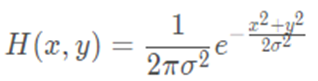不同尺寸的滤波器，得到的值也不同，下面是 (2k+1)x(2k+1) 滤波器的计算公式 :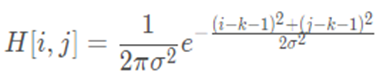常见的高斯滤波器大小为 5*5 σ = 1.4 ，其近似值为：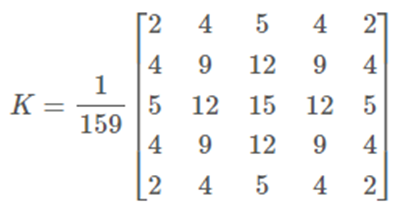# 计算梯度值和方向

常见方法采用 Sobel 滤波器 水平 x 和垂直 y 方向 在计算梯度和方向
采用下列公式计算梯度和方向：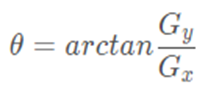# 非极大值抑制

目的：将模糊（blurred）的边界变得清晰（sharp）。通俗的讲，就是保留了每个像素点上梯度强度的极大值，而删掉其他的值。

•近邻 法：
a) 将其梯度方向近似为以下值中的一个（ 0,45,90,135,180,225,270,315 ）（即上下左右和 45 度方向）
b) 比较该像素点，和其梯度方向正负方向的像素点的梯度强度
c) 如果该像素点梯度强度最大则保留，否则抑制（删除，即置为 0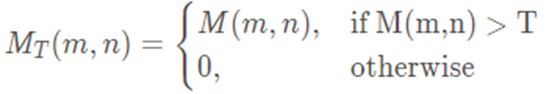•插值法：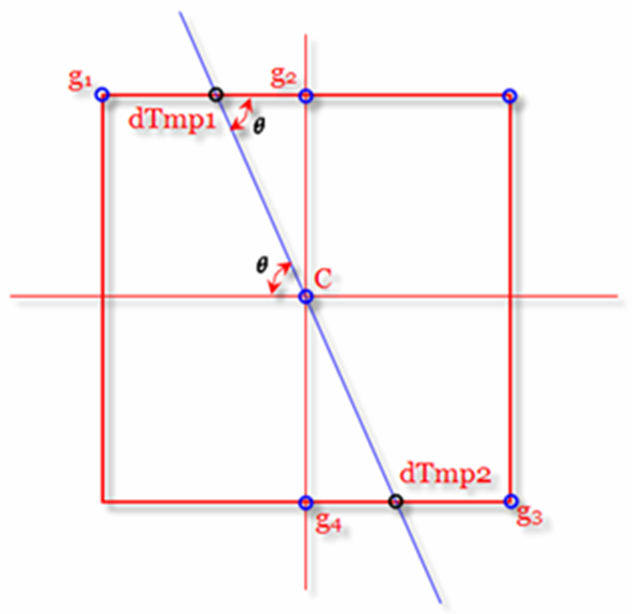# 双阈值的选取

使用双阈值来对二值化图像进行筛选，通过选取合适的大阈值与小阈值可以得出最为接近图像真实边缘的边缘图像。
实现方法：根据高阈值和低阈值将像素点标记为强边界点、弱边界点和非边界点三类。其中强边界点即为真实边缘点。

# 滞后边界跟踪

目的：进一步处理弱边界点
主要思想： 和强边界点相连的弱边界点认为是边界点，其他的弱边界点则被抑制。
方法：  由真实边缘引起的弱边缘像素将连接到强边缘像素，而噪声响应未连接。为了跟踪边缘连接， 通过查看弱边缘像素及其 8 个邻域像素 ，只要其中一个为强边缘像素，则该弱边缘点就可以保留为真实的边缘。

java代码如下：

main程序

package img;

import javax.imageio.ImageIO;

import java.awt.image.BufferedImage;
import java.io.File;

public class main {

public static void main(String[] args) throws Exception {
// 读取图片
// Canny边缘检测
Canny c=new Canny();
BufferedImage cannyImg=c.getCannyImg(bufImage,0.08,0.4,2);
ImageIO.write(cannyImg, "JPEG", new File("cannyImg.jpg"));

System.out.println("Main: Successfully!");
}

}


Canny程序

package img;

import java.awt.Color;
import java.awt.image.BufferedImage;
import java.io.IOException;

public class Canny {

static int edge[][];
static int find[][];
static double vmax=0;
static BufferedImage cannyImg;

public Canny() {
// TODO Auto-generated constructor stub
}
public static BufferedImage getCannyImg(BufferedImage image,double a,double b,double g) throws IOException {
// TODO Auto-generated method stub
// 灰度化
BufferedImage grayImg=getGray(image);
// 高斯滤波
grayImg=filtering_Gaussian(grayImg, g);
// 计算梯度幅度值和梯度方向
// 非极大值抑制处理(插值法)
// 双阈值处理
double thresh=vmax*a;
double_threshold(nms, b*thresh, thresh);
// 边界跟踪处理
boundary_tracking();
BufferedImage cannyImg=showCannyImg();
return cannyImg;
}
private static BufferedImage getGray(BufferedImage image) {
// TODO Auto-generated method stub
int w = image.getWidth();
int h = image.getHeight();

BufferedImage grayImg= new BufferedImage(w,h,image.getType());

for(int x=0;x<w;x++)
for(int y=0;y<h;y++) {
int pixel=image.getRGB(x, y);
int r=(pixel & 0xff0000) >> 16;
int g=(pixel & 0xff00) >> 8;
int b=(pixel & 0xff);
int gray = (int) (0.299 * r + 0.587 * g + 0.114 * b);
grayImg.setRGB(x, y, new Color(gray,gray,gray).getRGB());
}

return grayImg;
}
private static int RGBTogray(int pixel) {
// TODO Auto-generated method stub
return pixel & 0xff;
}
private static BufferedImage filtering_Gaussian(BufferedImage image, double g) {
// TODO Auto-generated method stub
int w = image.getWidth();
int h = image.getHeight();

int length=5;
int k=length/2;
double sigma=Math.sqrt(g);

double[][] gaussian=new double[length][length];
double sum=0;
for(int i=0;i<length;i++) {
for(int j=0;j<length;j++) {
gaussian[i][j]=Math.exp(-((i-k)*(i-k)+(j-k)*(j-k))/(2*sigma*sigma));
gaussian[i][j]/=2*Math.PI*sigma*sigma;
sum+=gaussian[i][j];
}
}
for(int i=0;i<length;i++) {
for(int j=0;j<length;j++) {
gaussian[i][j]/=sum;
}
}

BufferedImage GaussianImg= new BufferedImage(w,h,image.getType());

for(int x=k;x<w-k;x++) {
for(int y=k;y<h-k;y++) {
int newpixel=0;
for(int gx=0;gx<length;gx++) {
for(int gy=0;gy<length;gy++) {
int ix=x+gx-k;
int iy=y+gy-k;
if(ix<0||iy<0||ix>=w||iy>=h)
continue;
else {
newpixel+=RGBTogray(image.getRGB(ix, iy))*gaussian[gx][gy];
}
}
}
newpixel=(int) Math.round(1.0*newpixel);
GaussianImg.setRGB(x, y, new Color(newpixel,newpixel,newpixel).getRGB());
}
}
return GaussianImg;
}
// TODO Auto-generated method stub
int w = image.getWidth();
int h = image.getHeight();
int step=1;
for(int x=step;x<w-step;x++) {
for(int y=step;y<h-step;y++) {
int gx=-RGBTogray(image.getRGB(x-step, y-step))
-RGBTogray(image.getRGB(x-step, y))*2
-RGBTogray(image.getRGB(x-step, y+step))
+RGBTogray(image.getRGB(x+step, y-step))
+RGBTogray(image.getRGB(x+step, y))*2
+RGBTogray(image.getRGB(x+step, y+step));
int gy=RGBTogray(image.getRGB(x-step, y+step))
+RGBTogray(image.getRGB(x, y+step))*2
+RGBTogray(image.getRGB(x+step, y+step))
-RGBTogray(image.getRGB(x-step, y-step))
-RGBTogray(image.getRGB(x, y-step))*2
-RGBTogray(image.getRGB(x+step, y-step));
if(gx==0)
else
}
}

}
// TODO Auto-generated method stub
double[][] nms=new double[w][h];
for(int x=1;x<w-1;x++) {
for(int y=1;y<h-1;y++) {
continue;
if(a==-2||a==2) {
nms[x][y]=0;
}
else if(a==-1) {
nms[x][y]=0;
}
else if(a==0) {
nms[x][y]=0;
}
else if(a==1) {
nms[x][y]=0;
}
if(nms[x][y]>vmax)vmax=nms[x][y];
}
}

return nms;
}
private static double[][] NMS_2(double[][][] grad) {
// TODO Auto-generated method stub
double[][] nms=new double[w][h];
for(int x=1;x<w-1;x++) {
for(int y=1;y<h-1;y++) {
continue;
int x1,y1,x2,y2;
x1=0;y1=1;x2=1;y2=1;
weight=1.0/weight;
x1=1;y1=0;x2=1;y2=1;
weight*=1;
x1=1;y1=0;x2=1;y2=-1;
weight*=-1;
}else {
x1=0;y1=-1;x2=1;y2=-1;
weight=-1.0/weight;
}
nms[x][y]=0;
if(nms[x][y]>vmax)vmax=nms[x][y];
}
}
return nms;
}
private static void double_threshold(double[][] nms,double low_th,double high_th){
// TODO Auto-generated method stub
int w=nms.length;
int h=nms.length;
edge=new int[w][h];
for(int x=0;x<w;x++) {
for(int y=0;y<h;y++) {
if(nms[x][y]>=high_th) {
edge[x][y]=2;
}else if(nms[x][y]>=low_th) {
edge[x][y]=1;
}
}
}
}
private static void dfs(int x,int y,int w,int h) {
// TODO Auto-generated method stub
if(x<0||x>=w||y<0||y>=h||find[x][y]==1)return ;
find[x][y]=1;
if(edge[x][y]>=1) {
edge[x][y]=2;
for(int i=-1;i<=1;i++) {
for(int j=-1;j<=1;j++) {
dfs(x+i,y+j,w,h);
}
}
}
}
private static int[][] boundary_tracking(){
// TODO Auto-generated method stub
int w=edge.length;
int h=edge.length;

find=new int[w][h];

for(int x=0;x<w;x++) {
for(int y=0;y<h;y++) {
if(find[x][y]==1)continue;
if(edge[x][y]==2) {
dfs(x,y,w,h);
}else if(edge[x][y]==0) {
find[x][y]=1;
}
}
}
return edge;
}
private static BufferedImage showCannyImg() throws IOException {
// TODO Auto-generated method stub
int w=edge.length;
int h=edge.length;

BufferedImage cannyImg= new BufferedImage(w,h,BufferedImage.TYPE_INT_RGB);
for(int x=0;x<w;x++) {
for(int y=0;y<h;y++) {
if(edge[x][y]==2) {
cannyImg.setRGB(x, y, new Color(255,255,255).getRGB());
}else {
cannyImg.setRGB(x, y, new Color(0,0,0).getRGB());
}
}
}
return cannyImg;
}
}


处理结果展开全文计算机视觉
• import java.util.Arrays; import java.util.Comparator; import java.util.Scanner; /* * 如果要求最大值的话，那么每次先用最小的连个数相乘的结果+1， * 相反，如果要求最小值的话，每次先用最大的两个数相乘...

代码：

package com.hh;

import java.util.Arrays;
import java.util.Comparator;
import java.util.Scanner;

/*
* 如果要求最大值的话，那么每次先用最小的连个数相乘的结果+1，
* 相反，如果要求最小值的话，每次先用最大的两个数相乘的结果+1.
*/
public class SequenceMaxAndMin {
static Integer m[] =new Integer;
static Integer n[]=new Integer;
static Comparator<Integer> cmp = new Comparator<Integer>() {
public int compare(Integer i1, Integer i2) {
return i2-i1;
}
};
public static void main(String args[]) {
System.out.println("输入数组长度：");
Scanner sc=new Scanner(System.in);
int a=0;
a=sc.nextInt();
System.out.println("输入数组元素：");
for(int i=0;i<a;i++) {
m[i]=sc.nextInt();
n[i]=m[i];			}
//升序排序
Arrays.parallelSort(m,0,a);
for(int i=1;i<a;i++){
m[i]=m[i]*m[i-1]+1;//从小到大排完序之后，先从前边最小的两个数相乘然后存放到数组里
Arrays.parallelSort(m,i,a);/*算法的核心部分，由于每次从最小的两个数相乘后的数值
放到原来的数组中有可能会破坏数组从小到大的顺序,
所以再用一个sort排序，使新的数组变得有序,依次循环下去，就会得到最大值。*/
}

int max=m[a-1];
System.out.println("max"+max);
Arrays.sort(n,cmp);//逆序排序
for(Integer a1:n) {
System.out.println(a1);
}
for(int i=1;i<a;i++){
n[i]=n[i-1]*n[i]+1;/*而求最小值的时候就不用sort每次都排序啦，因为前边两个最大的数乘起来
的数不可能会比后边小的数还要小，所以求最小值得时候会比上边要简单一些*/
}

int min=n[a-1];
System.out.println("min:"+min);
System.out.println("极差为："+(max-min));
}

}



测试结果：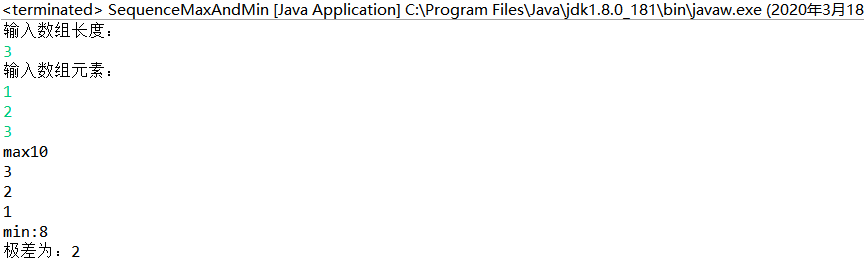展开全文• ## 数列极差-贪心算法

千次阅读 2016-11-10 22:52:19
进行如下操作：在数列中删除其中两个数a和b，然后在数列中的加入一个数a×b+1，如此下去，直至剩下一个数，在所有按这种操作方式最后得到的数中，最大的数记做Max，最小的数记做Min，则该数列的极差定义为M=Max-...
package com.work.home_3;

import java.util.Arrays;

/**
* 贪心算法-数列极差问题
* 说明：对于给定的数列，如果按数列中的值从小到大的顺序做运算，则可以得到数列的最大值；
* 如果按数列中的值从大到小做运算，则可得到数列的最小值。因此，对于给定的数列，先对数列进行排序，然后再进行最大、最小值的计算
*
* @author jun
*/
public class Greedy {
/*
* 贪心算法
*/
public static int greedy(int[] arr) {
// 对数组arr按升序进行排序
Arrays.sort(arr);
System.out.println("\n排序后数列值变为：");
System.out.println(Arrays.toString(arr) + "\n");
// 数列最大值
int max = arr;
// 数列最小值
int min = arr[arr.length - 1];
for (int i = 1, j = (arr.length - 2); i < arr.length || j >= 0; i++, j--) {
max = max * arr[i] + 1;
min = arr[j] * min + 1;
}
System.out.println("最大值 max = " + max);
System.out.println("最小值 min = " + min);
// 极差
return (max - min);
}
}

package com.work.home_3;

import java.util.Arrays;
import java.util.Scanner;
/**
* 测试函数
* @author jun
*
*/
public class Test {
public static void main(String[] args) {
Scanner sc = new Scanner(System.in);
System.out.println("请输入正整数数列的元素个数 n = ");
int n = sc.nextInt();
sc.close();
// 测试数组
int[] arr = new int[n];
// 给数组赋值
System.out.println("原始数列值为：");
for (int i = 0; i < arr.length; i++) {
arr[i] = (int) (Math.random() * 10);
}
// 输出数组值
System.out.println(Arrays.toString(arr));
//输出数列的极差
System.out.println("\n数列arr的极差为：" + Greedy.greedy(arr));
}
}

展开全文• JDK中的java.util.zip.GZIPInputStream / GZIPOutputStream便是这个算法的实现。 deflate，zip文件用的就是这一算法。与gzip的不同之处在于，你可以指定算法 的压缩级别，这样你可以在压缩时间和输出文件大小上进行...压缩 snappy gzip 性能测试
• 相关性算法----皮尔逊算法的实现，内涵java实现版本和python实现版本，已测。
•  * 贪心算法-数列极差问题  * 1. 问题描述：  * N个正数数列，进行如下操作：每一次擦去其中2个数，设a和b，然后在数列中加入一个数ab+1，如此下去直至只剩下一个数。  * 在所有按这种操作方式最后得到的数中...
• 针对当前经典中值滤波算法不足，提出一种最近邻像素值的大概率滤波算法（GPF），该算法将掩膜窗覆盖下的所有像素值视为一个像素值集，并定义评价各个元素对整个集合的影响度关系，同时提供计算这种影响度的方法，...
• 贪心算法-数列极差问题 【题目描述】   在黑板上写了N个正整数做成的一个数列，进行如下操作：每一次擦去其中的两个数a和b，然后在数列中加入一个数a×b+1，如此下去直至黑板上剩下一个数，在所有按这种操作...
• 哈希算法的原理及其 Java 实现一、目的二、运行环境三、基本原理及步骤（I）各种加密算法的原理：① DES 数据加密标准（Data Encryption Standard）：算法介绍算法流程优点缺点破解方式适用场景安全性② 3DES（DES ...
• ## (java)五大常用算法

万次阅读 多人点赞 2018-08-31 10:50:15
算法一：分治法 基本概念 1.把一个复杂的问题分成两个或更多的相同或相似的子问题，再把子问题分成更小的子问题……直到最后子问题可以简单的直接求解，原问题的解即子问题的解的合并。 2.分治策略是对于一个规模...
• 深入分析Java垃圾收集算法和垃圾收集器前言如何确定无效对象引用计数法(Reference Counting)可达性分析算法(Reachability Analysis)GC Root引用的分类强引用(Strong Reference)软引用(Soft Reference)弱引用(Weak ...jvm GCRoot
• 该系统使用java编写的基于用户的协同过滤算法（UserCF） 利用统计学的相关系数经常皮尔森（pearson）相关系数计算相关系数来实现千人千面的推荐系统。 协同过滤推荐算法是诞生最早，并且较为著名的推荐算法。主要的...
• **资源限制时间限制：1.0s 内存限制：1.0GB 问题描述　给定 N 个整数，请你找出这 N 个数中最大的那个和最小的那个。 输入格式　 ...import java.util.Arrays; import java.util.Scanner; public class Main
• 算法的学习对于培养一个人的逻辑思维能力是有大帮助的，它可以培养 我们养成思考分析问题，解决问题的能力。 如果一个算法有缺陷，或不适合某个问题，执行这个算法将不会解决这个问题。不同的算法可能用不同的时间...基础
• 4-13 数列极差问题 问题描述 在黑板上写了 N 个正数组成的一个数列，进行如下操作:每一次擦去其中 2 个数设为 a 和 b，然后在数列中加入一个数 a*b+1，如此下去直至黑板上只剩下一个数。在所有按这种 操作方式...
• 道格拉斯-普克抽稀算法过滤经纬度-java package com.wm.weather.backend.utils; import javax.swing.*; import java.text.DecimalFormat; import java.util.ArrayList; import java.util.List; public class Douglas...
• 欢迎关注专栏：Java架构技术进阶。里面有大量batj面试题集锦，还有各种技术分享，如有好文章也欢迎投稿哦。...字节跳动Java面试题精选——算法与数据结构【含答案】，点赞，转发，收藏一气呵成！ ...
• 求和、平均值、众数、中位数、中列数、四分位数、极差、四分位数、截断均值、方差、绝对平均差（AAD）、中位数绝对偏差、标准差 的数学方法 package cn.javacodes.utils; import java.util.Arrays; import java....
• ## java实现常见查找算法

万次阅读 多人点赞 2017-06-15 22:04:44
// 利用Java工具类Arrays构造长度为f[k]的新数组并指向引用a a = Arrays.copyOf(a, f[k]); // 对新数组后面多余的元素赋值最大的元素 for (int i = high + 1; i [k]; i++) { a[i] = a[high];//当key是是最大值...斐波那契查找
• · · · kmp算法是一种字符串匹配算法，用于在一个文本串中查找模式串的位置，出现的次数等；其中求解next数组是核心(只与模式串有关)，若记模式串为p，next[i] = j 表示p[i]之前的子串中，存在长度为j的相同前缀和...c++ python
• 计算机最重要32算法、机器学习10大经典算法、机器学习10大经典算法
• 排序是一个非常常见的应用场景，也是开发岗位面试必问的一道面试题，有人说，如果一个企业招聘开发人员的题目中没有排序算法题，那说明这个企业不是一个“正规”的企业，哈哈，虽然有点戏谑，但是也从侧面证明了排序...
• 贪婪算法： 在每一个阶段，可以认为所做出的的决定是最好的，而不考虑将来的后果。通常，这意味着选择的是某个局部最优。当算法终止时，我们希望局部最优等于全局最优，如果是这样的话，这个算法就是正确的，否则...Huffman
• 一致性哈希算法（Consistent Hashing）最早在论文《Consistent Hashing and Random Trees: Distributed Caching Protocols for Relieving Hot Spots on the World Wide Web》中被提出。简单来说，一致性哈希将整个...一致性hash
• 缺点是容错性、灵活性和对环境的适应性较，与各受控机器人存在通讯瓶颈问题。相对于集中式结构，分散式结构无法得到全局最优解，但它凭借着可靠性、灵活性和较强的环境适应性越来越受到广泛的青睐。分散式结构中的...python 人工智能 编程语言
• ## Java 查找算法

万次阅读 2015-07-08 19:49:15
但是因为寻找插入位置的查找操作的复杂度跟树的高度相关为logn，极差的情况下可能接近于线性查找。 平衡二叉树 平衡二叉树是尽量减少数高的二叉树，其算法中增加了左旋和右旋的操作。插入复杂度会高一些，...
• JAVA推荐系统系统原理该系统使用java编写的基于用户的协同过滤算法(UserCF)利用统计学的相关系数经常皮尔森(pearson)相关系数计算相关系数来实现千人千面的推荐系统。协同过滤推荐算法是诞生最早，并且较为著名的...
• 坐标形式）能够简化计算， 算法描述如下： function getNumberInNormalDistribution(mean,std_dev){ return mean+(randomNormalDistribution()* std_dev); } function randomNormalDistribution......